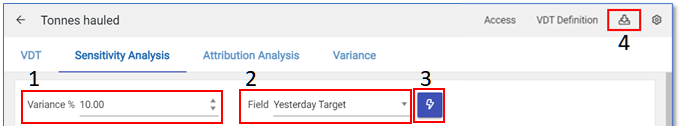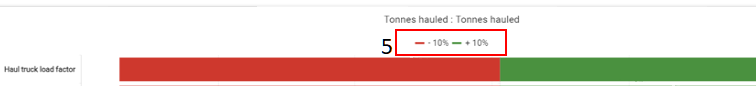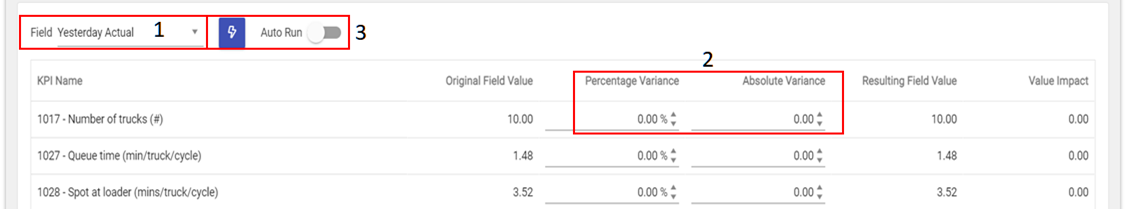Sensitivity Analysis

The Sensitivity Analysis report shows which input KPI’s have the biggest impact on a VDT’s outcome value. This is calculated by adjusting each KPI value by a fixed percentage and comparing the effect this has on the VDT result to that of the other KPIs.

To access the analysis, click on the Sensitivity Analysis tab.

1. The percentage value entered is applied to all KPI’s in the VDT, in order to ascertain the impact that increasing and decreasing the KPI value by this amount would have on the output value.
2. The selected field’s value will be used as the base value for impact calculations.
3. Re-runs the report using the percentage and field values that are currently entered.
4. Exports the report data to Excel format.
5. Series on the chart can be toggled on/off via the legendNote: The red/green colour coding indicates if plus or minus percentages are applied, not the effect on the output node (this is indicated by the side of the tornado chart). For example: If the distance is increased it will decrease the tonnes hauled, so there would be a green bar on the left side of the tornado chart.

The table below contains the variance values applied and the resulting net impact on the VDT result.The Attribution Analysis report compares the values of 2 fields across all KPI’s, in order to determine what the impact of substituting the one with the other would be on the VDT outcome.

To access the analysis, click on the Attribution Analysis tab.

1. The selected Control Field will be used as the base value for all calculations.
2. The selected Analysis Field values will be applied one by one for each KPI, in order to see what the impact on the VDT’s output node of moving from the base (Control) value to this (Analysis) value would be (for each KPI in isolation). To see what KPIs caused us to miss/exceed the target then use the target as the Control (base) field and the actual as of the Analysis Field.
• The control button between the fields (1) Control Field & (2) Analysis Field swaps the selected values around.
3. Run the report to update the chart and table.
4. The “Impact” series indicates the net impact of moving from the control value to the analysis value.
5. The “Delta Percentage” series indicates the difference in value between the control field and the target field, expressed as a percentage.
6. Hover over a bar to see more details.Variance

The Variance Analysis report allows the user to select individual percentage or absolute variance values per input KPI and then calculates the impact of this on the VDT outcome.

To access the analysis, click on the Variance tab.

1. The selected field’s values will be used as the base values for the report.
2. Variance percentage / absolute values can be set per KPI using the input box.
3. Run the report using the current input values and generate the resulting value impact, or select AutoRun to update the values after every edit.